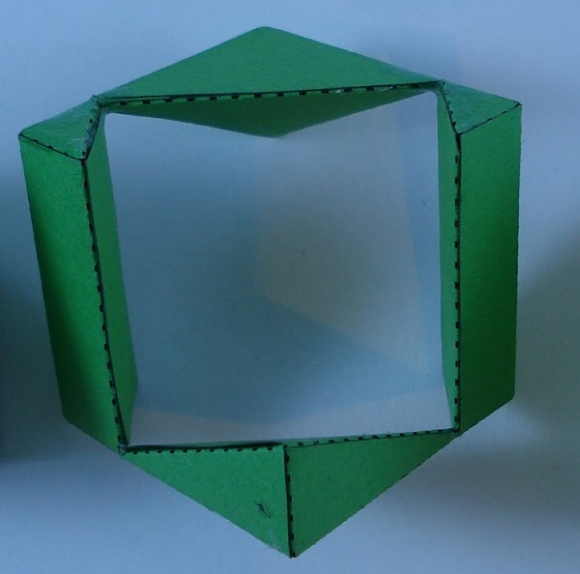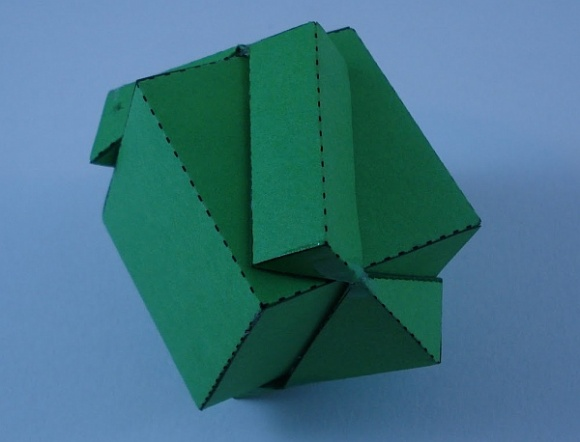### Math Monday: Passing a Cube Through Another Cube

by George Hart

It is an amazing fact that it’s mathematically possible to pass a cube through a hole in another cube of the same size. If you stand a cube up on a corner (with a diagonal vertical) and shine a light down on it, its shadow is a regular hexagon. If you calculate carefully, you’ll find that a square face of the cube can fit inside this hexagon with a bit of room to spare on all sides. This means that it is possible to drill a square hole through the cube in the direction of a diagonal and the outside parts will barely connect together. Martin Raynsford has made a template for a paper model that illustrates this idea. Here is the paper cube with a square hole:You’ll need to assemble it carefully. If you look at it from the side, you see there isn’t much material to hold itself together:The image below shows one cube passing through the other.Full disclosure requires me to point out the inner cube here is actually 2% smaller than the outer cube. Martin made it this way because otherwise he found that the thin bits of remaining paper tear. But rest assured that with mathematically ideal cubes and paper, not only could the two cubes be the same size, but you can actually pass a cube through a hole in a slightly smaller cube!